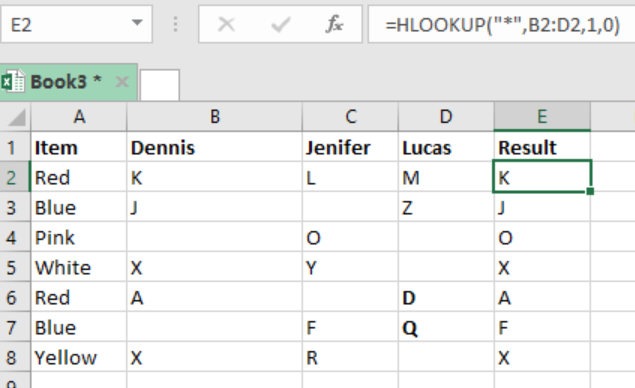Get instant live expert help with Excel or Google Sheets“My Excelchat expert helped me in less than 20 minutes, saving me what would have been 5 hours of work!”

#### Post your problem and you’ll get Expert help in seconds.

Your message must be at least 40 characters
Our professional Expert are available now. Your privacy is guaranteed.

# How to Get the First Text Value with HLOOKUP

The HLOOKUP function is excel is fundamental when it comes to looking up and retrieving a text value in a given range of columns. This article looks at how one can get the first text value with HLOOKUP.Figure 1: How to find first text value in a list in excel

## General syntax of the formula

`=HLOOKUP(“*”, range, 1, FALSE)`

## Understanding the formula

We use the HLOOKUP function when we want to get the first text value of a text string.

## How the formula works

• The HLOOKUP function is fundamental when it comes to extracting the first text value when given text strings in several columns.
• The HLOOKUP function, together with the asterisk wildcard character (*) can help us in this
• In the example above, we have the formula in cell E2 as `=HLOOKUP("*",B2:D2,1,0)`
• Here, the wildcard value is the “*” which matches one or more text values.
• To get the first text for the other columns in the table array, we need to copy down the formula down column E.
• Given that we have only one row in the range, the row index number is 1.
• We set the range lookup value to zero, which implies FALSE, to force exact match.
• The function will find and return the first text value in each row that is found in column B through D.

## Instant Connection to an Expert through our Excelchat Service

Most of the time, the problem you will need to solve will be more complex than a simple application of a formula or function. If you want to save hours of research and frustration, try our live Excelchat service! Our Excel Experts are available 24/7 to answer any Excel question you may have. We guarantee a connection within 30 seconds and a customized solution within 20 minutes.

### Did this post not answer your question? Get a solution from connecting with the expert.Another blog reader asked this question today on Excelchat:## Subscribe to Excelchat.coAnother blog reader asked this question today on Excelchat: